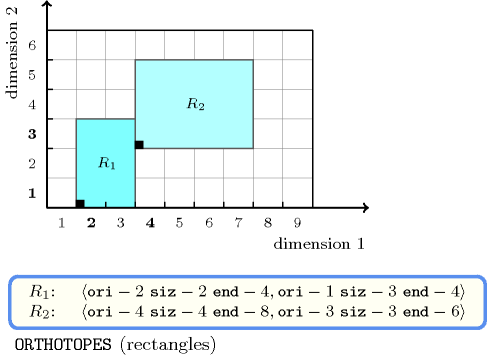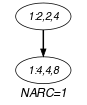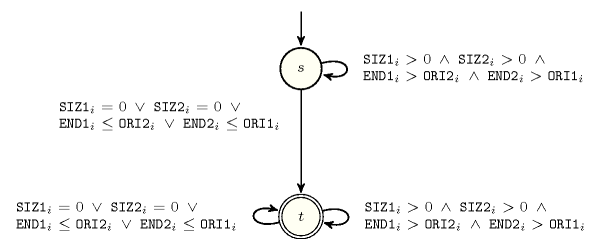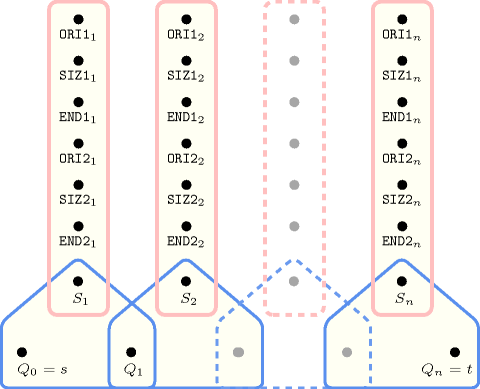## 5.410. two_orth_do_not_overlap

Origin

Used for defining $\mathrm{𝚍𝚒𝚏𝚏𝚗}$.

Constraint

$\mathrm{𝚝𝚠𝚘}_\mathrm{𝚘𝚛𝚝𝚑}_\mathrm{𝚍𝚘}_\mathrm{𝚗𝚘𝚝}_\mathrm{𝚘𝚟𝚎𝚛𝚕𝚊𝚙}\left(\mathrm{𝙾𝚁𝚃𝙷𝙾𝚃𝙾𝙿𝙴}\mathtt{1},\mathrm{𝙾𝚁𝚃𝙷𝙾𝚃𝙾𝙿𝙴}\mathtt{2}\right)$

Type
 $\mathrm{𝙾𝚁𝚃𝙷𝙾𝚃𝙾𝙿𝙴}$ $\mathrm{𝚌𝚘𝚕𝚕𝚎𝚌𝚝𝚒𝚘𝚗}\left(\mathrm{𝚘𝚛𝚒}-\mathrm{𝚍𝚟𝚊𝚛},\mathrm{𝚜𝚒𝚣}-\mathrm{𝚍𝚟𝚊𝚛},\mathrm{𝚎𝚗𝚍}-\mathrm{𝚍𝚟𝚊𝚛}\right)$
Arguments
 $\mathrm{𝙾𝚁𝚃𝙷𝙾𝚃𝙾𝙿𝙴}\mathtt{1}$ $\mathrm{𝙾𝚁𝚃𝙷𝙾𝚃𝙾𝙿𝙴}$ $\mathrm{𝙾𝚁𝚃𝙷𝙾𝚃𝙾𝙿𝙴}\mathtt{2}$ $\mathrm{𝙾𝚁𝚃𝙷𝙾𝚃𝙾𝙿𝙴}$
Restrictions
 $|\mathrm{𝙾𝚁𝚃𝙷𝙾𝚃𝙾𝙿𝙴}|>0$ $\mathrm{𝚛𝚎𝚚𝚞𝚒𝚛𝚎}_\mathrm{𝚊𝚝}_\mathrm{𝚕𝚎𝚊𝚜𝚝}$$\left(2,\mathrm{𝙾𝚁𝚃𝙷𝙾𝚃𝙾𝙿𝙴},\left[\mathrm{𝚘𝚛𝚒},\mathrm{𝚜𝚒𝚣},\mathrm{𝚎𝚗𝚍}\right]\right)$ $\mathrm{𝙾𝚁𝚃𝙷𝙾𝚃𝙾𝙿𝙴}.\mathrm{𝚜𝚒𝚣}\ge 0$ $\mathrm{𝙾𝚁𝚃𝙷𝙾𝚃𝙾𝙿𝙴}.\mathrm{𝚘𝚛𝚒}\le \mathrm{𝙾𝚁𝚃𝙷𝙾𝚃𝙾𝙿𝙴}.\mathrm{𝚎𝚗𝚍}$ $|\mathrm{𝙾𝚁𝚃𝙷𝙾𝚃𝙾𝙿𝙴}\mathtt{1}|=|\mathrm{𝙾𝚁𝚃𝙷𝙾𝚃𝙾𝙿𝙴}\mathtt{2}|$ $\mathrm{𝚘𝚛𝚝𝚑}_\mathrm{𝚕𝚒𝚗𝚔}_\mathrm{𝚘𝚛𝚒}_\mathrm{𝚜𝚒𝚣}_\mathrm{𝚎𝚗𝚍}$$\left(\mathrm{𝙾𝚁𝚃𝙷𝙾𝚃𝙾𝙿𝙴}\mathtt{1}\right)$ $\mathrm{𝚘𝚛𝚝𝚑}_\mathrm{𝚕𝚒𝚗𝚔}_\mathrm{𝚘𝚛𝚒}_\mathrm{𝚜𝚒𝚣}_\mathrm{𝚎𝚗𝚍}$$\left(\mathrm{𝙾𝚁𝚃𝙷𝙾𝚃𝙾𝙿𝙴}\mathtt{2}\right)$
Purpose

For two orthotopes ${O}_{1}$ and ${O}_{2}$ enforce that there exists at least one dimension $i$ such that the projections on $i$ of ${O}_{1}$ and ${O}_{2}$ do not overlap.

Example
$\left(\begin{array}{c}〈\mathrm{𝚘𝚛𝚒}-2\mathrm{𝚜𝚒𝚣}-2\mathrm{𝚎𝚗𝚍}-4,\mathrm{𝚘𝚛𝚒}-1\mathrm{𝚜𝚒𝚣}-3\mathrm{𝚎𝚗𝚍}-4〉,\hfill \\ 〈\mathrm{𝚘𝚛𝚒}-4\mathrm{𝚜𝚒𝚣}-4\mathrm{𝚎𝚗𝚍}-8,\mathrm{𝚘𝚛𝚒}-3\mathrm{𝚜𝚒𝚣}-3\mathrm{𝚎𝚗𝚍}-6〉\hfill \end{array}\right)$

Figure 5.410.1 represents the respective position of the two rectangles of the example. The coordinates of the leftmost lowest corner of each rectangle are stressed in bold. The $\mathrm{𝚝𝚠𝚘}_\mathrm{𝚘𝚛𝚝𝚑}_\mathrm{𝚍𝚘}_\mathrm{𝚗𝚘𝚝}_\mathrm{𝚘𝚟𝚎𝚛𝚕𝚊𝚙}$ constraint holds since the two rectangles do not overlap.

##### Figure 5.410.1. The two non overlapping rectangles of the Example slotTypical
$|\mathrm{𝙾𝚁𝚃𝙷𝙾𝚃𝙾𝙿𝙴}|>1$
Symmetries
• Arguments are permutable w.r.t. permutation $\left(\mathrm{𝙾𝚁𝚃𝙷𝙾𝚃𝙾𝙿𝙴}\mathtt{1},\mathrm{𝙾𝚁𝚃𝙷𝙾𝚃𝙾𝙿𝙴}\mathtt{2}\right)$.

• Items of $\mathrm{𝙾𝚁𝚃𝙷𝙾𝚃𝙾𝙿𝙴}\mathtt{1}$ and $\mathrm{𝙾𝚁𝚃𝙷𝙾𝚃𝙾𝙿𝙴}\mathtt{2}$ are permutable (same permutation used).

• $\mathrm{𝙾𝚁𝚃𝙷𝙾𝚃𝙾𝙿𝙴}\mathtt{1}.\mathrm{𝚜𝚒𝚣}$ can be decreased to any value $\ge 0$.

• $\mathrm{𝙾𝚁𝚃𝙷𝙾𝚃𝙾𝙿𝙴}\mathtt{2}.\mathrm{𝚜𝚒𝚣}$ can be decreased to any value $\ge 0$.

Used in
Keywords
Arc input(s)

$\mathrm{𝙾𝚁𝚃𝙷𝙾𝚃𝙾𝙿𝙴}\mathtt{1}$ $\mathrm{𝙾𝚁𝚃𝙷𝙾𝚃𝙾𝙿𝙴}\mathtt{2}$

Arc generator
$\mathrm{𝑆𝑌𝑀𝑀𝐸𝑇𝑅𝐼𝐶}_\mathrm{𝑃𝑅𝑂𝐷𝑈𝐶𝑇}$$\left(=\right)↦\mathrm{𝚌𝚘𝚕𝚕𝚎𝚌𝚝𝚒𝚘𝚗}\left(\mathrm{𝚘𝚛𝚝𝚑𝚘𝚝𝚘𝚙𝚎}\mathtt{1},\mathrm{𝚘𝚛𝚝𝚑𝚘𝚝𝚘𝚙𝚎}\mathtt{2}\right)$

Arc arity
Arc constraint(s)
$\mathrm{𝚘𝚛𝚝𝚑𝚘𝚝𝚘𝚙𝚎}\mathtt{1}.\mathrm{𝚎𝚗𝚍}\le \mathrm{𝚘𝚛𝚝𝚑𝚘𝚝𝚘𝚙𝚎}\mathtt{2}.\mathrm{𝚘𝚛𝚒}\vee \mathrm{𝚘𝚛𝚝𝚑𝚘𝚝𝚘𝚙𝚎}\mathtt{1}.\mathrm{𝚜𝚒𝚣}=0$
Graph property(ies)
$\mathrm{𝐍𝐀𝐑𝐂}$$\ge 1$

Graph class
 $•$$\mathrm{𝙱𝙸𝙿𝙰𝚁𝚃𝙸𝚃𝙴}$ $•$$\mathrm{𝙽𝙾}_\mathrm{𝙻𝙾𝙾𝙿}$

Graph model

We build an initial graph where each arc corresponds to the fact that, either the projection of an orthotope on a given dimension is empty, either it is located before the projection in the same dimension of the other orthotope. Finally we ask that at least one arc constraint remains in the final graph.

Parts (A) and (B) of Figure 5.410.2 respectively show the initial and final graph associated with the Example slot. Since we use the $\mathrm{𝐍𝐀𝐑𝐂}$ graph property, the unique arc of the final graph is stressed in bold. It corresponds to the fact that the projection in dimension 1 of the first orthotope is located before the projection in dimension 1 of the second orthotope. Therefore the two orthotopes do not overlap.

##### Figure 5.410.2. Initial and final graph of the $\mathrm{𝚝𝚠𝚘}_\mathrm{𝚘𝚛𝚝𝚑}_\mathrm{𝚍𝚘}_\mathrm{𝚗𝚘𝚝}_\mathrm{𝚘𝚟𝚎𝚛𝚕𝚊𝚙}$ constraint(a) (b)
Automaton

Figure 5.410.3 depicts the automaton associated with the $\mathrm{𝚝𝚠𝚘}_\mathrm{𝚘𝚛𝚝𝚑}_\mathrm{𝚍𝚘}_\mathrm{𝚗𝚘𝚝}_\mathrm{𝚘𝚟𝚎𝚛𝚕𝚊𝚙}$ constraint. Let $\mathrm{𝙾𝚁𝙸}{\mathtt{1}}_{i}$, $\mathrm{𝚂𝙸𝚉}{\mathtt{1}}_{i}$ and $\mathrm{𝙴𝙽𝙳}{\mathtt{1}}_{i}$ respectively be the $\mathrm{𝚘𝚛𝚒}$, the $\mathrm{𝚜𝚒𝚣}$ and the $\mathrm{𝚎𝚗𝚍}$ attributes of the ${i}^{th}$ item of the $\mathrm{𝙾𝚁𝚃𝙷𝙾𝚃𝙾𝙿𝙴}\mathtt{1}$ collection. Let $\mathrm{𝙾𝚁𝙸}{\mathtt{2}}_{i}$, $\mathrm{𝚂𝙸𝚉}{\mathtt{2}}_{i}$ and $\mathrm{𝙴𝙽𝙳}{\mathtt{2}}_{i}$ respectively be the $\mathrm{𝚘𝚛𝚒}$, the $\mathrm{𝚜𝚒𝚣}$ and the $\mathrm{𝚎𝚗𝚍}$ attributes of the ${i}^{th}$ item of the $\mathrm{𝙾𝚁𝚃𝙷𝙾𝚃𝙾𝙿𝙴}\mathtt{2}$ collection. To each sextuple $\left(\mathrm{𝙾𝚁𝙸}{\mathtt{1}}_{i},\mathrm{𝚂𝙸𝚉}{\mathtt{1}}_{i},\mathrm{𝙴𝙽𝙳}{\mathtt{1}}_{i},\mathrm{𝙾𝚁𝙸}{\mathtt{2}}_{i},\mathrm{𝚂𝙸𝚉}{\mathtt{2}}_{i},\mathrm{𝙴𝙽𝙳}{\mathtt{2}}_{i}\right)$ corresponds a 0-1 signature variable ${S}_{i}$ as well as the following signature constraint: $\left(\left(\mathrm{𝚂𝙸𝚉}{\mathtt{1}}_{i}>0\right)\wedge \left(\mathrm{𝚂𝙸𝚉}{\mathtt{2}}_{i}>0\right)\wedge \left(\mathrm{𝙴𝙽𝙳}{\mathtt{1}}_{i}>\mathrm{𝙾𝚁𝙸}{\mathtt{2}}_{i}\right)\wedge \left(\mathrm{𝙴𝙽𝙳}{\mathtt{2}}_{i}>\mathrm{𝙾𝚁𝙸}{\mathtt{1}}_{i}\right)\right)⇔{S}_{i}$.

##### Figure 5.410.3. Automaton of the $\mathrm{𝚝𝚠𝚘}_\mathrm{𝚘𝚛𝚝𝚑}_\mathrm{𝚍𝚘}_\mathrm{𝚗𝚘𝚝}_\mathrm{𝚘𝚟𝚎𝚛𝚕𝚊𝚙}$ constraint##### Figure 5.410.4. Hypergraph of the reformulation corresponding to the automaton of the $\mathrm{𝚝𝚠𝚘}_\mathrm{𝚘𝚛𝚝𝚑}_\mathrm{𝚍𝚘}_\mathrm{𝚗𝚘𝚝}_\mathrm{𝚘𝚟𝚎𝚛𝚕𝚊𝚙}$ constraint